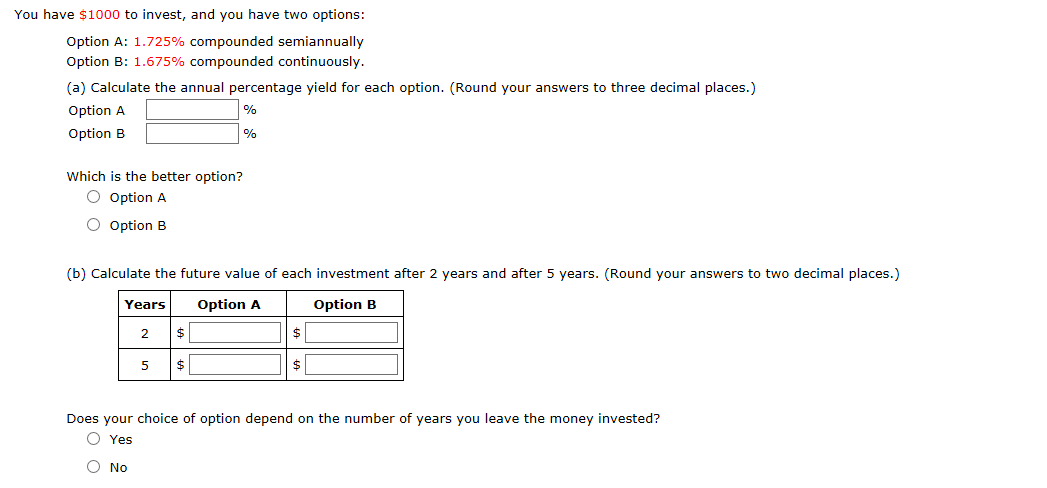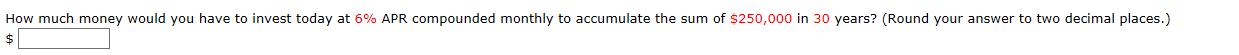# You have $1000 to invest,, and you have two options: Option A: 1.725% compounded semiannually Option B: 1.675% compounded continuously. (a) Calculate the annual percentage yield for each option. (Round your answers to three decimal places.) Option A % Option B % Which is the better option? O Option A O Option B (b) Calculate the future value of each investment after 2 years and after 5 years. (Round your answers to two decimal places.) Years Option A Option B$ 2  Does your choice of option depend on the number of years you leave the money invested? O Yes How much money would you have to invest today at 6% APR compounded monthly to accumulate the sum of $250,000 in 30 years? (Round your answer to two decimal places.) Questionhelp_outlineImage TranscriptioncloseYou have$1000 to invest,, and you have two options: Option A: 1.725% compounded semiannually Option B: 1.675% compounded continuously. (a) Calculate the annual percentage yield for each option. (Round your answers to three decimal places.) Option A % Option B % Which is the better option? O Option A O Option B (b) Calculate the future value of each investment after 2 years and after 5 years. (Round your answers to two decimal places.) Years Option A Option B $2$ $Does your choice of option depend on the number of years you leave the money invested? O Yes fullscreenhelp_outlineImage TranscriptioncloseHow much money would you have to invest today at 6% APR compounded monthly to accumulate the sum of$250,000 in 30 years? (Round your answer to two decimal places.) fullscreen

## Expert Answer

### Want to see this answer and more?

Experts are waiting 24/7 to provide step-by-step solutions in as fast as 30 minutes!*

*Response times may vary by subject and question complexity. Median response time is 34 minutes for paid subscribers and may be longer for promotional offers.
Tagged in
Math
Calculus© 2021 bartleby. All Rights Reserved.